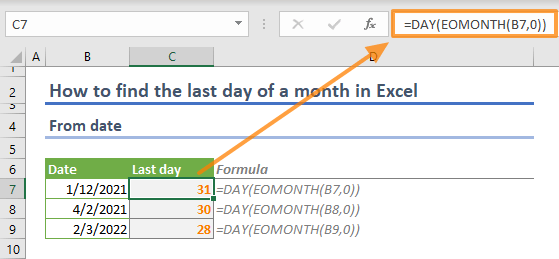In this article, we are going to show you how to find the last day of a month in Excel.

We will be using two functions to find the last day of a month in Excel: DAY and EOMONTH.

=DAY(EOMONTH(<Date>,0))

The EOMONTH returns the date value of the last day of the month, which is a certain number of months before or after the entered date. Per its definition, the function gets a date and a number of months as input. Zero (0) months means the function will look only into the same month as the date entered in the other argument. Thus, the following formula returns the date of the last day of the month.

EOMONTH(<Date>,0)

Next, you need to parse the outcome from the result date. You can use the DAY function for this. The only input for the DAY function is the date value.

DAY(<Date>)## Find the last day of a month from month number

In a scenario where you have several months whose last days you want to find in the current year, the DAY and the EOMONTH functions can be used like in the previous example. However, you will also need the DATE function here to generate the date value for EOMONTH.

The date function returns a date value for the given year, month, and day numbers. Below is the syntax:

DATE(<year>,<month>,<day>)

You will need to enter the month and year values. You can either supply these values yourself, or use the TODAY and YEAR functions to populate the current year dynamically.

The TODAY function returns the present day, and the YEAR returns the year number of the given date, just like in the DAY function.

The present year: =YEAR(TODAY())

If we combine these functions to find the last day of a month, we will have something like below:

=DAY(EOMONTH(DATE(YEAR(TODAY()),<number of month>,1),0))